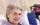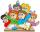# Square

If we increase one side of square by its one-half then square perimeter increase by 10 cm.

What is the side of the square?

Result

a =  5 cm

#### Solution:Leave us a comment of example and its solution (i.e. if it is still somewhat unclear...):Be the first to comment!#### To solve this example are needed these knowledge from mathematics:

Do you have a linear equation or system of equations and looking for its solution? Or do you have quadratic equation?

## Next similar examples:

1. RhombusIf we increase the rhombus side by 2.4 cm; its perimeter is 20 cm. How long was the side of the rhombus?
2. Unknown number 11That number increased by three equals three times itself?
3. CakesGrandmother baked cakes. Half of its was poppy, quarter with plum jam and 16 cheesecakes. How many cakes she baked in total?
4. Find xSolve: if 2(x-1)=14, then x= (solve an equation with one unknown)
5. Dropped sheetsThree consecutive sheets dropped from the book. The sum of the numbers on the pages of the dropped sheets is 273. What number has the last page of the dropped sheets?
6. Negative in equation2x + 3 + 7x = – 24, what is the value of x?
7. Forest nurseryIn the forest nursery after winter, they found that 1/10 stems died out of them. For them, they land 193 new spruces. How many spruces are in the forest nursery?
8. Simple equationSolve for x: 3(x + 2) = x - 18
9. Equation?
10. Unknown numberIdentify unknown number which 1/5 is 40 greater than one tenth of that number.
11. One-thirdA one-third of unknown number is equal to five times as great as the difference of the same unknown number and number 28. Determine the unknown number.
12. EquationSolve the equation: 1/2-2/8 = 1/10; Write the result as a decimal number.
13. NormThree workers planted 3555 seedlings of tomatoes in one dey. First worked at the standard norm, the second planted 120 seedlings more and the third 135 seedlings more than the first worker. How many seedlings were standard norm?
14. Equation 29Solve next equation: 2 ( 2x + 3 ) = 8 ( 1 - x) -5 ( x -2 )
15. Simple equationSolve the following simple equation: 2. (4x + 3) = 2-5. (1-x)
16. FamilyMom is 39 years old. Karl is eight years and Paul 4 years. For many years mother would old just like two children together?
17. ClassroomIn a class are 32 pupils. Boys are 8 less than girls. How many boys and girls are in the classroom?